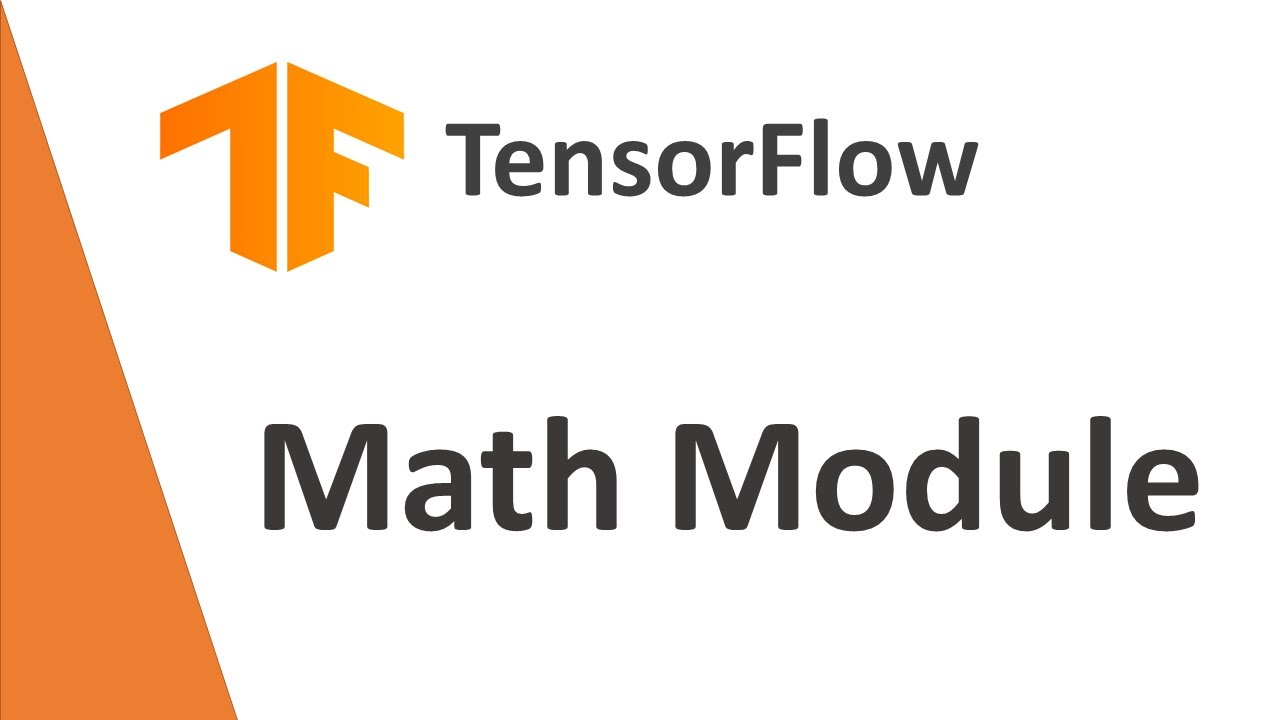# TensorFlow Tutorial : Math Module in TensorFlowIn this video we will learn the TensorFlow Math Module

In this video we will learn the TensorFlow Math Module

## 5 Steps to Passing the TensorFlow Developer Certificate

In this tutorial, we'll learn 5 Steps to Passing the TensorFlow Developer Certificate. Deep Learning is one of the most in demand skills on the market and TensorFlow is the most popular DL Framework.

## What is TensorFlow? TensorFlow

An end-to-end open-source platform for Machine Learning. Before we start with TensorFlow, we will need to know what machine learning and deep learning technologies are.

## Serving TensorFlow models with TensorFlow Serving

This is a detailed guide on how to create TensorFlow models and then deploy them using TensorFlow Serving

## A TensorFlow Modeling Pipeline using TensorFlow Datasets and TensorBoard

This article investigates TensorFlow components for building a toolset to make modeling evaluation more efficient. Specifically, TensorFlow Datasets (TFDS) and TensorBoard (TB) can be quite helpful in this task.

## Keras vs. Tensorflow - Difference Between Tensorflow and Keras

Keras vs Tensorflow - Learn the differences between Keras and Tensorflow on basis of Ease to use, Fast development,Functionality,flexibility,Performance etc